# Question 7 Which of the following is an equation of an ellipse? x² + 2y² =...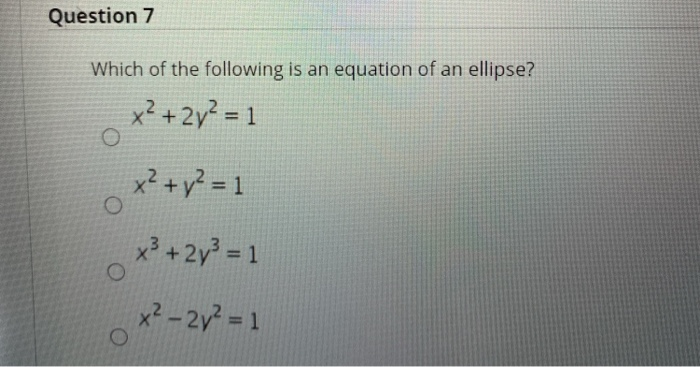Question 7 Which of the following is an equation of an ellipse? x² + 2y² = 1 x² + y² = 1 x3 + 2y3 = 1 O x² – 2y² = 1

Please comment if you need any clarification.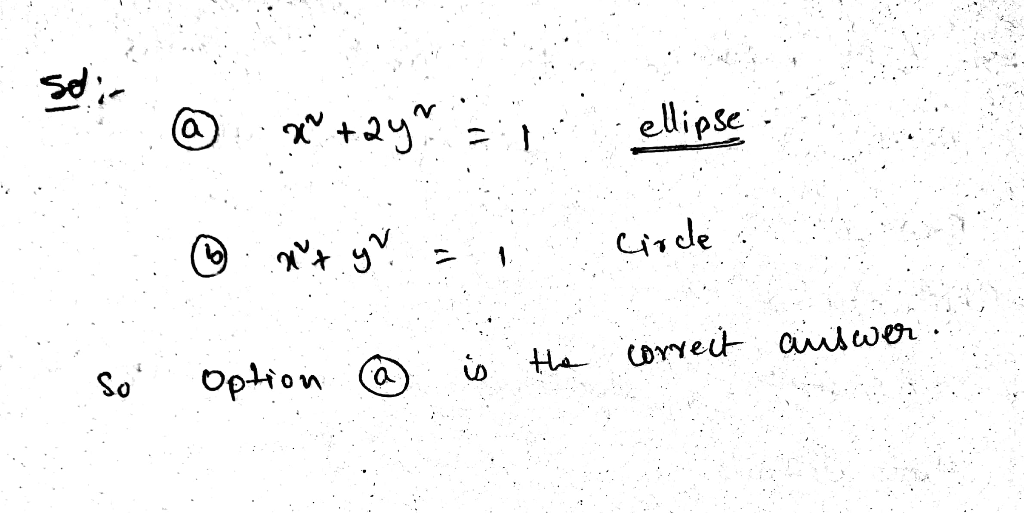##### Add Answer of: Question 7 Which of the following is an equation of an ellipse? x² + 2y² =...
Similar Homework Help Questions
• ### Question 7 Which of the following is an equation of an ellipse? X2 + 2y2 =...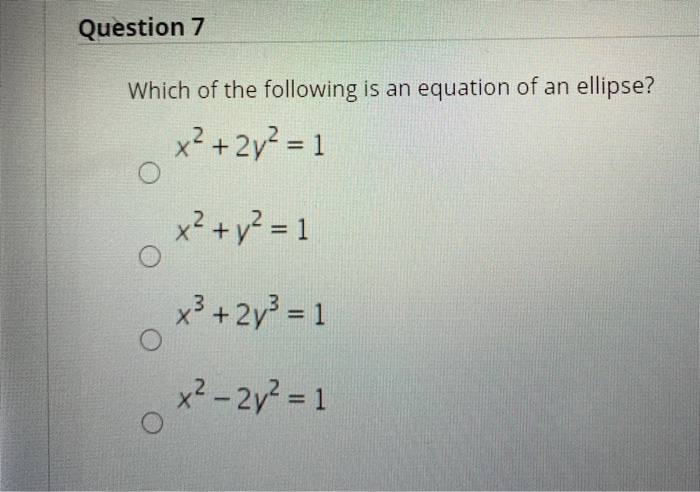Question 7 Which of the following is an equation of an ellipse? X2 + 2y2 = 1 x2 + y2 = 1 O x²+2y3 = 1 x2 - 2y2 = 1 O Question 8 Which of the following is equivalent to 14x-1|<11? (You are not asked to solve the inequality.) 4x-1<11, or 4x - 1>- 11 -11<4x-1<11 O 4x-1<11 -11 > 4x - 1<11 Question 9 Which of the following is equivalent to logxy? logy - logx Iny - Inx...

• ### I want to double check my answer regarding the following math problem: Write an equation in the slope intercept form if possible: for the line which is at (0,-3) and is perpendicular to x - 2y = 7 My equation is: x-2y=7 x=2y+7 2y+y=x 2y/2 = x/2

I want to double check my answer regarding the following math problem: Write an equation in the slope intercept form if possible: for the line which is at (0,-3) and is perpendicular to x - 2y = 7 My equation is: x-2y=7 x=2y+7 2y+y=x 2y/2 = x/2 - 7/2 Y intercept is x/2 - 7/2 Now I'm lost as far as the slope is concerned Please help

• ### Question 7 3 pts The solution of the Initial-Value Problem (IVP) x? yll – 2y =...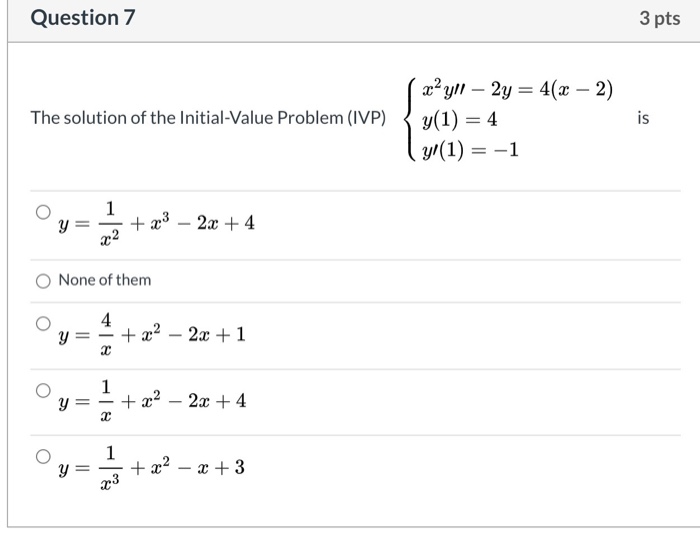Question 7 3 pts The solution of the Initial-Value Problem (IVP) x? yll – 2y = 4(x - 2) y(1) = 4 yl(1) = -1 is 1 y = + x3 - 2x + 4 22 None of them 4 y = + x2 23 + 1 2 1 O Y + x2 – 2x + 4 2 O y = *+2-- + x2 - x + 3 23

• ### Question 7 of 20 : Select the best answer for the question. 7. Find the standard...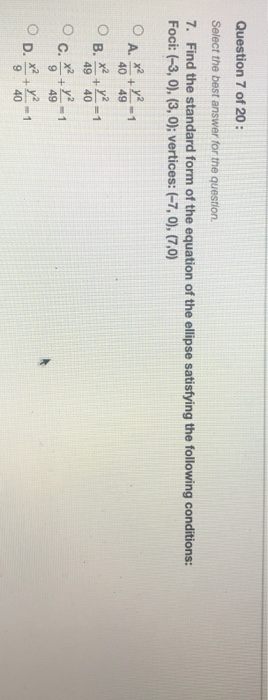Question 7 of 20 : Select the best answer for the question. 7. Find the standard form of the equation of the ellipse satisfying the following conditions: Foci: (-3,0), (3, 0); vertices: (-7, 0), (7,0) O A. x² ² + 40 49 O B. x2 + y2 = 1 49 40 O c. x2 + y2 - 1 9 49 D. x² + y² 9 40 -1

• ### Show that the ellipse (x^2)/(a^2)+2y^2 = 1 ?

Show that the ellipse #(x^2)/(a^2)+2y^2 #= 1 and the hyperbola #(x^2)/(a^2-1)##-2y^2#= 1 intersect at right angles.

• ### An ellipse equation

An ellipse has the equation ((x-2)^2/16)+((y-8)^2/49)=1Is the major axis vertical or horizontal?Find the exact values of the foci of the ellipse.

• ### An ellipse equation

An ellipse has the equation ((x-2)^2/16)+((y-8)^2/49)=1Is the major axis horizontal or vertical?Find the exact values of the foci of the ellipse

• ### Find the coordinates of the foci of an ellipse with equation:

**Note that the ^2 means squared.**7. Find the coordinates of the foci of an ellipse with equation:x^2 / 9 + y^2 / 16 = 1

• ### QUESTION 7 In the equation S-Y -T). , which of the following is an endogenous variable?...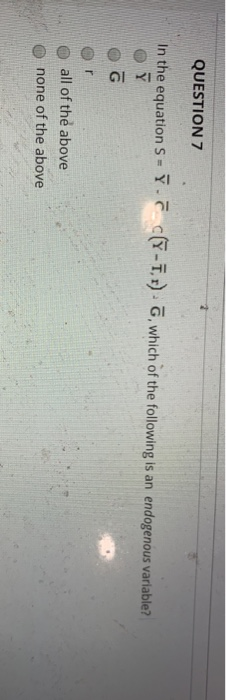QUESTION 7 In the equation S-Y -T). , which of the following is an endogenous variable? o all of the above o none of the above

• ### Question 10 of 20: Select the best answer for the question. 10. Identify the following equation...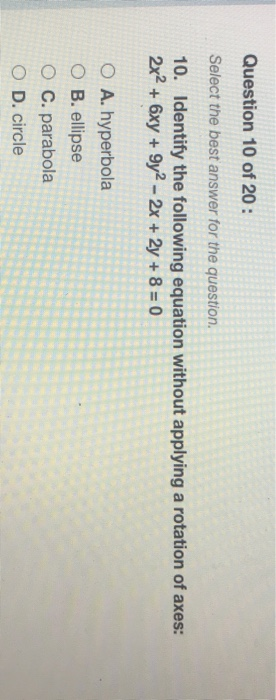Question 10 of 20: Select the best answer for the question. 10. Identify the following equation without applying a rotation of axes: 2x2 + 6xy +9y2 - 2x + 2y + 8 = 0 O A. hyperbola O B. ellipse O C. parabola O D. circle

Free Homework App